/scikit-learn

# sklearn.cluster.MiniBatchKMeans

`class sklearn.cluster.MiniBatchKMeans(n_clusters=8, init=’k-means++’, max_iter=100, batch_size=100, verbose=0, compute_labels=True, random_state=None, tol=0.0, max_no_improvement=10, init_size=None, n_init=3, reassignment_ratio=0.01)` [source]

Mini-Batch K-Means clustering

Read more in the User Guide.

Parameters: `n_clusters : int, optional, default: 8` The number of clusters to form as well as the number of centroids to generate. `init : {‘k-means++’, ‘random’ or an ndarray}, default: ‘k-means++’` Method for initialization, defaults to ‘k-means++’: ‘k-means++’ : selects initial cluster centers for k-mean clustering in a smart way to speed up convergence. See section Notes in k_init for more details. ‘random’: choose k observations (rows) at random from data for the initial centroids. If an ndarray is passed, it should be of shape (n_clusters, n_features) and gives the initial centers. `max_iter : int, optional` Maximum number of iterations over the complete dataset before stopping independently of any early stopping criterion heuristics. `batch_size : int, optional, default: 100` Size of the mini batches. `verbose : boolean, optional` Verbosity mode. `compute_labels : boolean, default=True` Compute label assignment and inertia for the complete dataset once the minibatch optimization has converged in fit. `random_state : int, RandomState instance or None (default)` Determines random number generation for centroid initialization and random reassignment. Use an int to make the randomness deterministic. See Glossary. `tol : float, default: 0.0` Control early stopping based on the relative center changes as measured by a smoothed, variance-normalized of the mean center squared position changes. This early stopping heuristics is closer to the one used for the batch variant of the algorithms but induces a slight computational and memory overhead over the inertia heuristic. To disable convergence detection based on normalized center change, set tol to 0.0 (default). `max_no_improvement : int, default: 10` Control early stopping based on the consecutive number of mini batches that does not yield an improvement on the smoothed inertia. To disable convergence detection based on inertia, set max_no_improvement to None. `init_size : int, optional, default: 3 * batch_size` Number of samples to randomly sample for speeding up the initialization (sometimes at the expense of accuracy): the only algorithm is initialized by running a batch KMeans on a random subset of the data. This needs to be larger than n_clusters. `n_init : int, default=3` Number of random initializations that are tried. In contrast to KMeans, the algorithm is only run once, using the best of the `n_init` initializations as measured by inertia. `reassignment_ratio : float, default: 0.01` Control the fraction of the maximum number of counts for a center to be reassigned. A higher value means that low count centers are more easily reassigned, which means that the model will take longer to converge, but should converge in a better clustering. `cluster_centers_ : array, [n_clusters, n_features]` Coordinates of cluster centers labels_ : Labels of each point (if compute_labels is set to True). `inertia_ : float` The value of the inertia criterion associated with the chosen partition (if compute_labels is set to True). The inertia is defined as the sum of square distances of samples to their nearest neighbor.

`KMeans`
The classic implementation of the clustering method based on the Lloyd’s algorithm. It consumes the whole set of input data at each iteration.

#### Examples

```>>> from sklearn.cluster import MiniBatchKMeans
>>> import numpy as np
>>> X = np.array([[1, 2], [1, 4], [1, 0],
...               [4, 2], [4, 0], [4, 4],
...               [4, 5], [0, 1], [2, 2],
...               [3, 2], [5, 5], [1, -1]])
>>> # manually fit on batches
>>> kmeans = MiniBatchKMeans(n_clusters=2,
...         random_state=0,
...         batch_size=6)
>>> kmeans = kmeans.partial_fit(X[0:6,:])
>>> kmeans = kmeans.partial_fit(X[6:12,:])
>>> kmeans.cluster_centers_
array([[1, 1],
[3, 4]])
>>> kmeans.predict([[0, 0], [4, 4]])
array([0, 1], dtype=int32)
>>> # fit on the whole data
>>> kmeans = MiniBatchKMeans(n_clusters=2,
...         random_state=0,
...         batch_size=6,
...         max_iter=10).fit(X)
>>> kmeans.cluster_centers_
array([[3.95918367, 2.40816327],
[1.12195122, 1.3902439 ]])
>>> kmeans.predict([[0, 0], [4, 4]])
array([1, 0], dtype=int32)
```

#### Methods

 `fit`(X[, y, sample_weight]) Compute the centroids on X by chunking it into mini-batches. `fit_predict`(X[, y, sample_weight]) Compute cluster centers and predict cluster index for each sample. `fit_transform`(X[, y, sample_weight]) Compute clustering and transform X to cluster-distance space. `get_params`([deep]) Get parameters for this estimator. `partial_fit`(X[, y, sample_weight]) Update k means estimate on a single mini-batch X. `predict`(X[, sample_weight]) Predict the closest cluster each sample in X belongs to. `score`(X[, y, sample_weight]) Opposite of the value of X on the K-means objective. `set_params`(**params) Set the parameters of this estimator. `transform`(X) Transform X to a cluster-distance space.
`__init__(n_clusters=8, init=’k-means++’, max_iter=100, batch_size=100, verbose=0, compute_labels=True, random_state=None, tol=0.0, max_no_improvement=10, init_size=None, n_init=3, reassignment_ratio=0.01)` [source]
`fit(X, y=None, sample_weight=None)` [source]

Compute the centroids on X by chunking it into mini-batches.

Parameters: `X : array-like or sparse matrix, shape=(n_samples, n_features)` Training instances to cluster. It must be noted that the data will be converted to C ordering, which will cause a memory copy if the given data is not C-contiguous. `y : Ignored` not used, present here for API consistency by convention. `sample_weight : array-like, shape (n_samples,), optional` The weights for each observation in X. If None, all observations are assigned equal weight (default: None)
`fit_predict(X, y=None, sample_weight=None)` [source]

Compute cluster centers and predict cluster index for each sample.

Convenience method; equivalent to calling fit(X) followed by predict(X).

Parameters: `X : {array-like, sparse matrix}, shape = [n_samples, n_features]` New data to transform. `y : Ignored` not used, present here for API consistency by convention. `sample_weight : array-like, shape (n_samples,), optional` The weights for each observation in X. If None, all observations are assigned equal weight (default: None) `labels : array, shape [n_samples,]` Index of the cluster each sample belongs to.
`fit_transform(X, y=None, sample_weight=None)` [source]

Compute clustering and transform X to cluster-distance space.

Equivalent to fit(X).transform(X), but more efficiently implemented.

Parameters: `X : {array-like, sparse matrix}, shape = [n_samples, n_features]` New data to transform. `y : Ignored` not used, present here for API consistency by convention. `sample_weight : array-like, shape (n_samples,), optional` The weights for each observation in X. If None, all observations are assigned equal weight (default: None) `X_new : array, shape [n_samples, k]` X transformed in the new space.
`get_params(deep=True)` [source]

Get parameters for this estimator.

Parameters: `deep : boolean, optional` If True, will return the parameters for this estimator and contained subobjects that are estimators. `params : mapping of string to any` Parameter names mapped to their values.
`partial_fit(X, y=None, sample_weight=None)` [source]

Update k means estimate on a single mini-batch X.

Parameters: `X : array-like, shape = [n_samples, n_features]` Coordinates of the data points to cluster. It must be noted that X will be copied if it is not C-contiguous. `y : Ignored` not used, present here for API consistency by convention. `sample_weight : array-like, shape (n_samples,), optional` The weights for each observation in X. If None, all observations are assigned equal weight (default: None)
`predict(X, sample_weight=None)` [source]

Predict the closest cluster each sample in X belongs to.

In the vector quantization literature, `cluster_centers_` is called the code book and each value returned by `predict` is the index of the closest code in the code book.

Parameters: `X : {array-like, sparse matrix}, shape = [n_samples, n_features]` New data to predict. `sample_weight : array-like, shape (n_samples,), optional` The weights for each observation in X. If None, all observations are assigned equal weight (default: None) `labels : array, shape [n_samples,]` Index of the cluster each sample belongs to.
`score(X, y=None, sample_weight=None)` [source]

Opposite of the value of X on the K-means objective.

Parameters: `X : {array-like, sparse matrix}, shape = [n_samples, n_features]` New data. `y : Ignored` not used, present here for API consistency by convention. `sample_weight : array-like, shape (n_samples,), optional` The weights for each observation in X. If None, all observations are assigned equal weight (default: None) `score : float` Opposite of the value of X on the K-means objective.
`set_params(**params)` [source]

Set the parameters of this estimator.

The method works on simple estimators as well as on nested objects (such as pipelines). The latter have parameters of the form `<component>__<parameter>` so that it’s possible to update each component of a nested object.

Returns: self
`transform(X)` [source]

Transform X to a cluster-distance space.

In the new space, each dimension is the distance to the cluster centers. Note that even if X is sparse, the array returned by `transform` will typically be dense.

Parameters: `X : {array-like, sparse matrix}, shape = [n_samples, n_features]` New data to transform. `X_new : array, shape [n_samples, k]` X transformed in the new space.

## Examples using `sklearn.cluster.MiniBatchKMeans`Biclustering documents with the Spectral Co-clustering algorithm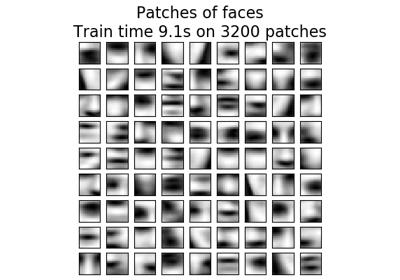Online learning of a dictionary of parts of faces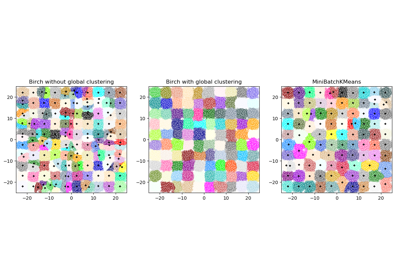Compare BIRCH and MiniBatchKMeans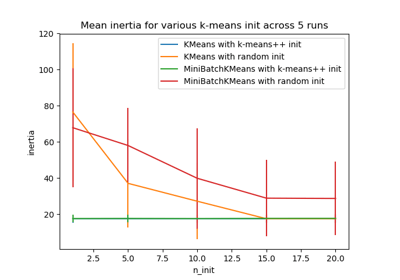Empirical evaluation of the impact of k-means initialization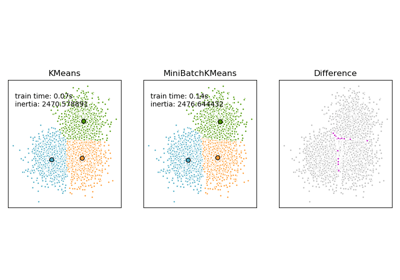Comparison of the K-Means and MiniBatchKMeans clustering algorithms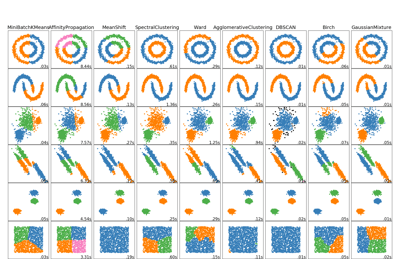Comparing different clustering algorithms on toy datasets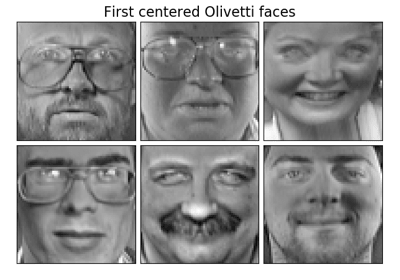Faces dataset decompositionsClustering text documents using k-means